Courses

Test: Construction Of Tangents

10 Questions MCQ Test Mathematics (Maths) Class 10 | Test: Construction Of Tangents

Description
Attempt Test: Construction Of Tangents | 10 questions in 10 minutes | Mock test for Class 10 preparation | Free important questions MCQ to study Mathematics (Maths) Class 10 for Class 10 Exam | Download free PDF with solutions
QUESTION: 1

In which of the following is AD not the bisector of angle A?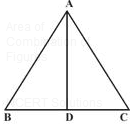​

Solution:

The angle bisector of an angle of a triangle divides the opposite side internally in the ratio of the sides containing the angle.So in this question,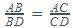So , Substituting the values of option C
LHS=5/1.5=1/0.5
RHS=10/3.5=2/0.7
So, RHS ≠ LHS

QUESTION: 2

Solution:
QUESTION: 3

A point O is at a distance of 10 cm from the centre of a circle of radius 6 cm. How many tangents can be drawn from point O to the circle?

Solution:

Only two tangents can be drawn from an external point.No tangent  can be drawn which touches points inside the circle.

QUESTION: 4

If TP and TQ are two tangents to a circle with centre O so that angle POQ = 110° then angle PTQ is equal to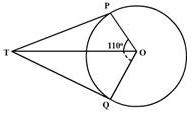Solution:

We know that the sum of angle made by two tangents joined by an exterior point and the angle made at the centre of the circle is equal to 180 degrees
So, Angle POQ + Angle PTQ = 180
110 + Angle PTQ = 180
Angle PTQ = 180- 110 = 70 degrees

QUESTION: 5

D and E are the mid points of sides AB and AC respectively of a triangle ABC such that DE is parallel to BC. If AD = x cm, DB = (x – 2) cm, AE = (x + 2) cm and EC = (x-1) cm, then the value of x is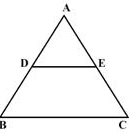Solution:
QUESTION: 6

PQ is a tangent to a circle with centre O at the point P. If triangle OPQ is an isosceles triangle, then angle OQP is equal to​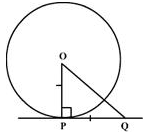Solution:
QUESTION: 7

To draw a pair of tangents to a circle which are inclined to each other at an angle of 60°, it is required to draw tangents at the end points to those two radii of the side, the angle between which is​

Solution:

∠OQP=90o=∠ORP since the angle, between a tangent to  a circle and the radius of the same circle passing through  the point of contact, is 90o

∴  By angle sum property of quadrilaterals, we get ∠OQP+∠RPQ+∠ORP+∠ROQ=360o⟹90o+60o+90o+∠ROQ=360o⟹∠ROQ=120o.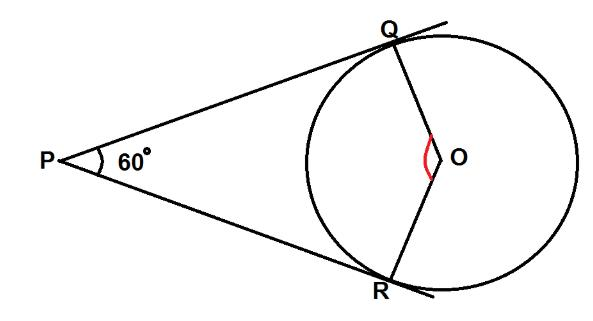QUESTION: 8

To draw a pair of tangents to a circle which are inclined to each other at an angle of 45° it is required to draw tangents at the end point of those two radii of the circle, the angle between which is :​

Solution:
QUESTION: 9

A point O is at a distance of 10 cm from the centre of a circle of radius 6 cm. How many tangents can be drawn from point O to the circle?

Solution:

Two tangents can be drawn to a circle from a point outside the circle.
No tangent line can be drawn through a point within a circle, since any such line must be a secant line.

QUESTION: 10

Length of the tangent to a circle from a point 26 cm away from the centre is 24 cm. What is the radius of the circle?​

Solution:Use Code STAYHOME200 and get INR 200 additional OFF Use Coupon Code

Track your progress, build streaks, highlight & save important lessons and more!

Similar ContentRelated tests